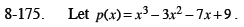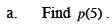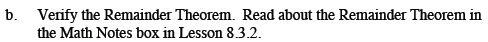### Home > CCA2 > Chapter Ch8 > Lesson 8.3.3 > Problem8-175

8-175.
1. Let p(x) = x3 − 3x2 − 7x + 9. Homework Help ✎

1. Find p(5).

2. Verify the Remainder Theorem. Read about the Remainder Theorem in the Math Notes box in Lesson 8.3.2.Substitute 5 in for x.$\frac{\textit{x}^3-3\textit{x}^2-7\textit{x}+9}{\textit{x}-5}=?$